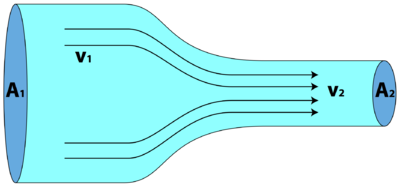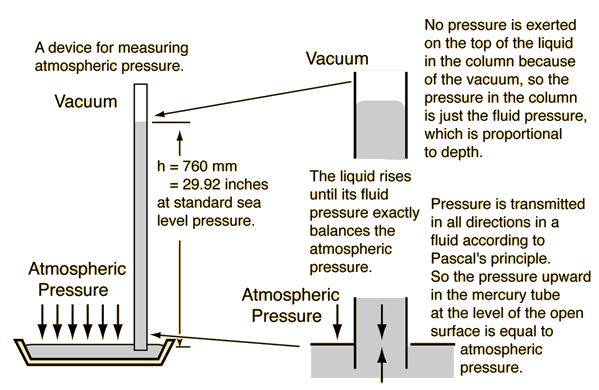Pressure

In other languages: EspañolFrançais

Pressure is an important concept in physics, and is defined as the magnitude of force acting perpendicular to a surface, per unit area. The SI unit for pressure is the pascal (Pa), defined as 1 newton per meter squared.

, where

• P is pressure
• F is the force applied perpendicular (normal) to the surface, and
• A is the area of the applied force.

An easy way to understand pressure is to imagine holding a tack, and pressing it lightly between forefinger and thumb, with the sharp end on the thumb and the head on the forefinger. The thumb will begin to feel pain immediately while the forefinger will not. The tack is exerting the same amount of force on both thumb and forefinger, but the pressure on the thumb is much larger because of the small area over which the force is applied.

Pressure is important in many physical applications; it is a key concept of fluid mechanics, used in the ideal gas law to describe the energy of a gas, and many more situations.

Fluid PressureFigure 1: A decrease in cross sectional area increases force in these parts which increases the fluids velocity (v2 is faster).

In fluid mechanics, pressure can create many useful devices in different situations. In plumbing, different cross-sectional areas for the pipes creates higher speeds at different locations, where smaller areas result in higher speeds (see Figure 1). This controls how fluids flow in rivers and hoses.

Pressure in fluids with no net motion, like the ocean or the atmosphere, dictates much of what happens to the fluids. The atmosphere is a compressible fluid (changes volume when pushed) made up of many different gases, so depending on where a person is on the Earth there will be a pressure acting on them (with pressure at sea level being defined as 1 atm).

For incompressible fluids like water, the relationship gives the pressure under a column of fluid, where is the density of a fluid, is the height, and is the acceleration of gravity. Instruments like the mercury barometer (see Figure 2) can be used to determine pressures from the atmosphere.Figure 2: Mercury barometer measuring atmospheric pressure.

Gas Pressure

Pressure is determined by the flow of mass from a region of high pressure to a region of low pressure, and this is most visible with gasses.

For example, a balloon being filled with air expands because the pressure inside the balloon is increasing to pressures higher than those outside of it. Since pressure is a property that determines which direction mass flows, as soon as the balloon is released the air moves from a region of high pressure to a region of low pressure and the balloon deflates.

This tendency of pressure to flow from high pressure regions to low pressure regions is the driving force of wind on the Earth. Due to uneven heating of the Earth, different areas are at higher pressures than others so this causes the flow of air in the atmosphere (shown in Figure 3). Most weather phenomena, from hurricanes to tornadoes, are a product of pressure and temperature, and are also influenced by the rotation of the Earth (see Coriolis effect).